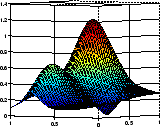Main Content

# franke

Franke's bivariate test function

## Syntax

```z = franke(x,y) ```

## Description

`z = franke(x,y) ` returns the value `z(i)` of Franke's function at the site (`x(i)`,`y(i)`), `i=1:numel(x)`, with `z` of the same size as `x` and `y` (which must be of the same size).

Franke's function is the following weighted sum of four exponentials:

## Examples

The following commands provide a plot of Franke's function:

```pts = (0:50)/50; [x,y] = ndgrid(pts,pts); z = franke(x,y); surf(x,y,z), view(145,-2), set(gca,'Fontsize',16) ```## References

 Richard Franke. “A critical comparison of some methods for interpolation of scattered data.” Naval Postgraduate School Tech.Rep. NPS-53-79-003, March 1979.

## Support

#### Machine Learning Challenges: Choosing the Best Classification Model and Avoiding Overfitting

Download white paper